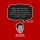Home
IT Knowledge
Inspiration
Languages
EN

# Python - generate random string of size n characters

0 points
Created by:Haris65
386

In this article, we would like to show you how to generate a random string of size n characters in Python.

Quick solution:

``````import string
from random import choice

N = 10
group = string.ascii_letters + string.digits

print("".join(choice(group) for i in range(N)))  # 2C9foB1rbe``````

## Practical example

In this example, we generate a random string consisting of letters (uppercase & lowercase) and digits.

``````import string
from random import choice

N = 10
group = string.ascii_letters + string.digits

print("".join(choice(group) for i in range(N)))  # 7ZTmrwjEwV``````

Output:

``7ZTmrwjEwV``

## Explanation

In this section, we want to explain step by step how to generate random strings in Python.

1. Choose from which group of characters you want to generate the random string. You can choose the group from the Character groups section below. Join them using `+` operator.
2. Use `choice()` function from `random` module with all the character groups.
3. Repeat character selection specified number of times (`N`) using `for` loop.
4. Join all characters to the empty string (`""`) using `join()` method.

## Character groups

You can choose the character groups from which you would like to get the characters.

• `string.ascii_letters`
• `string.ascii_lowercase`
• `string.ascii_uppercase`
• `string.letters`
• `string.lowercase`
• `string.uppercase`
• `string.digits`
• `string.hexdigits`
• `string.octdigits`
• `string.punctuation`
• `string.printable`
• `string.whitespace`

Note:

You can choose multiple groups using `+` operator.